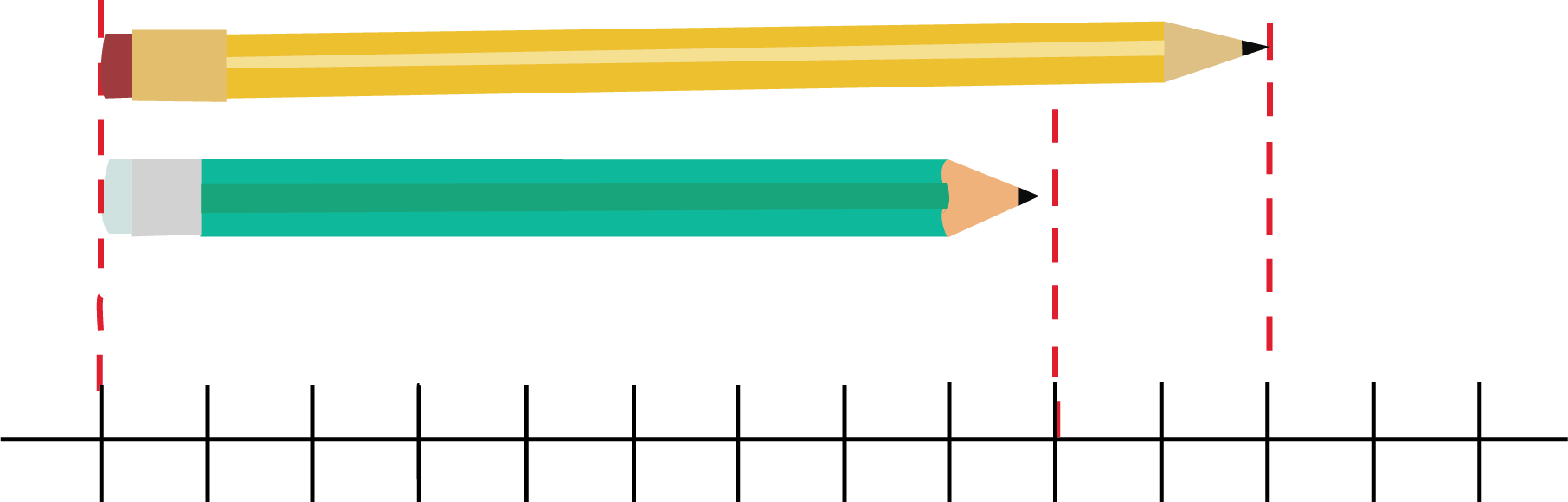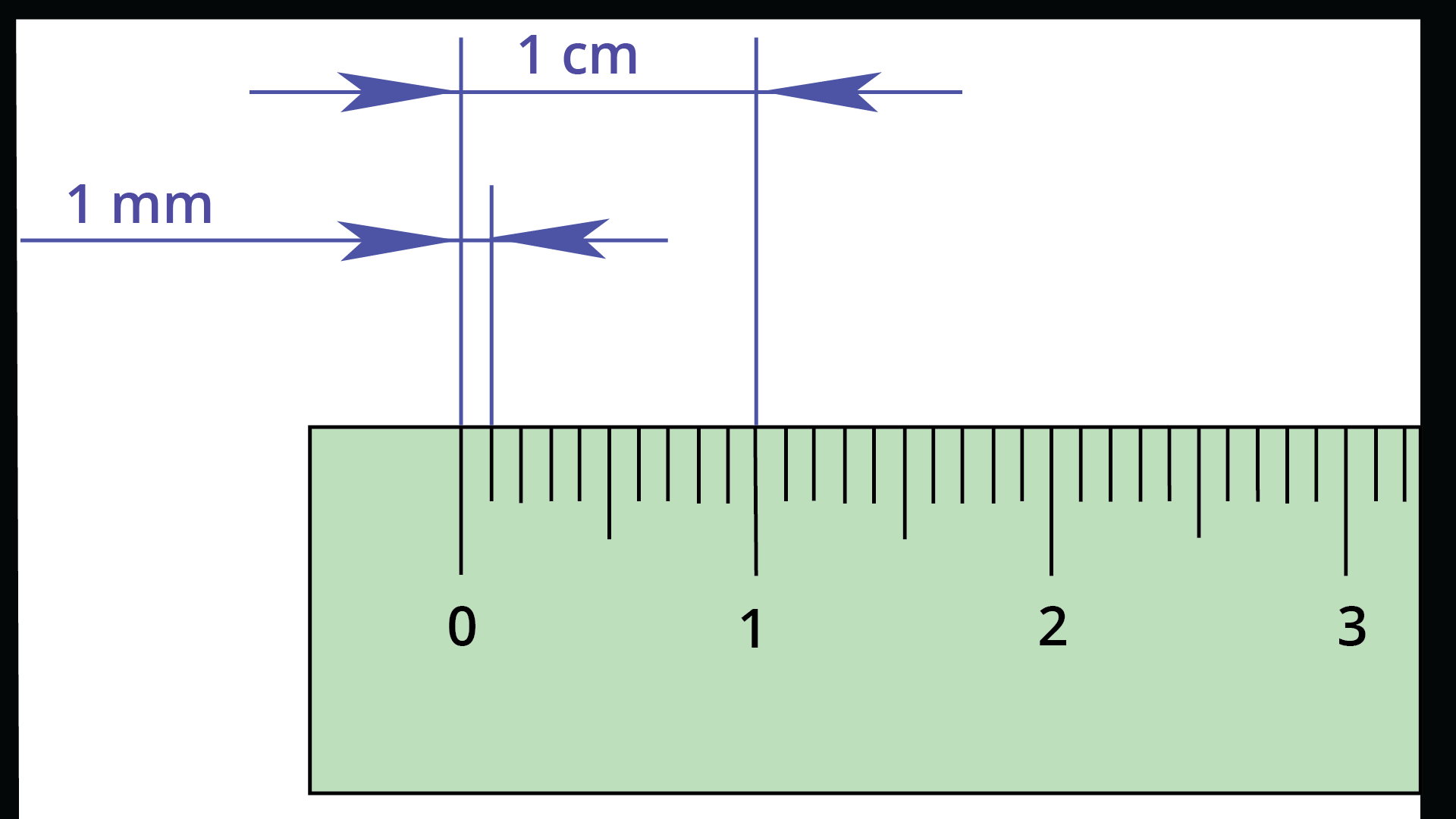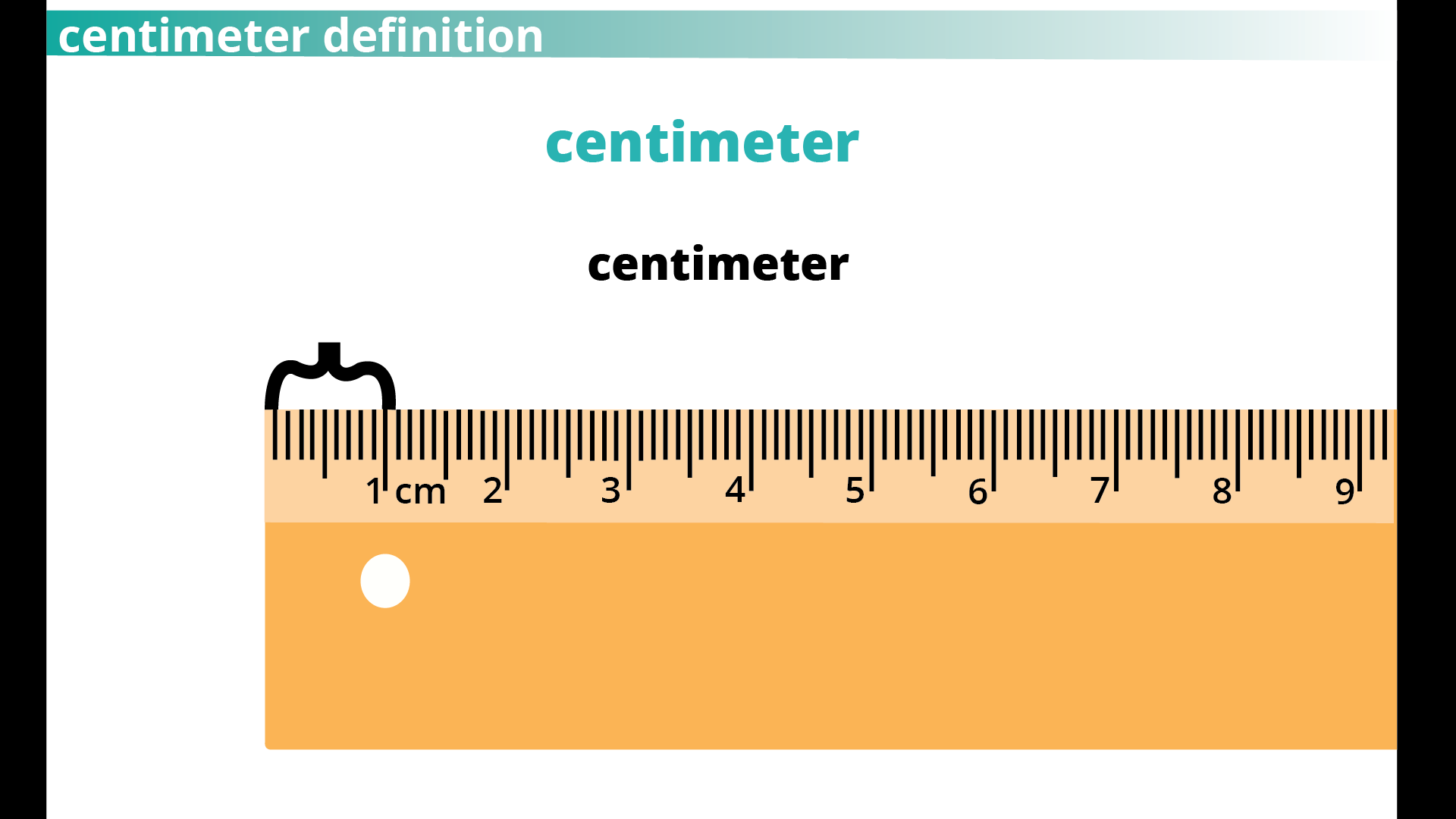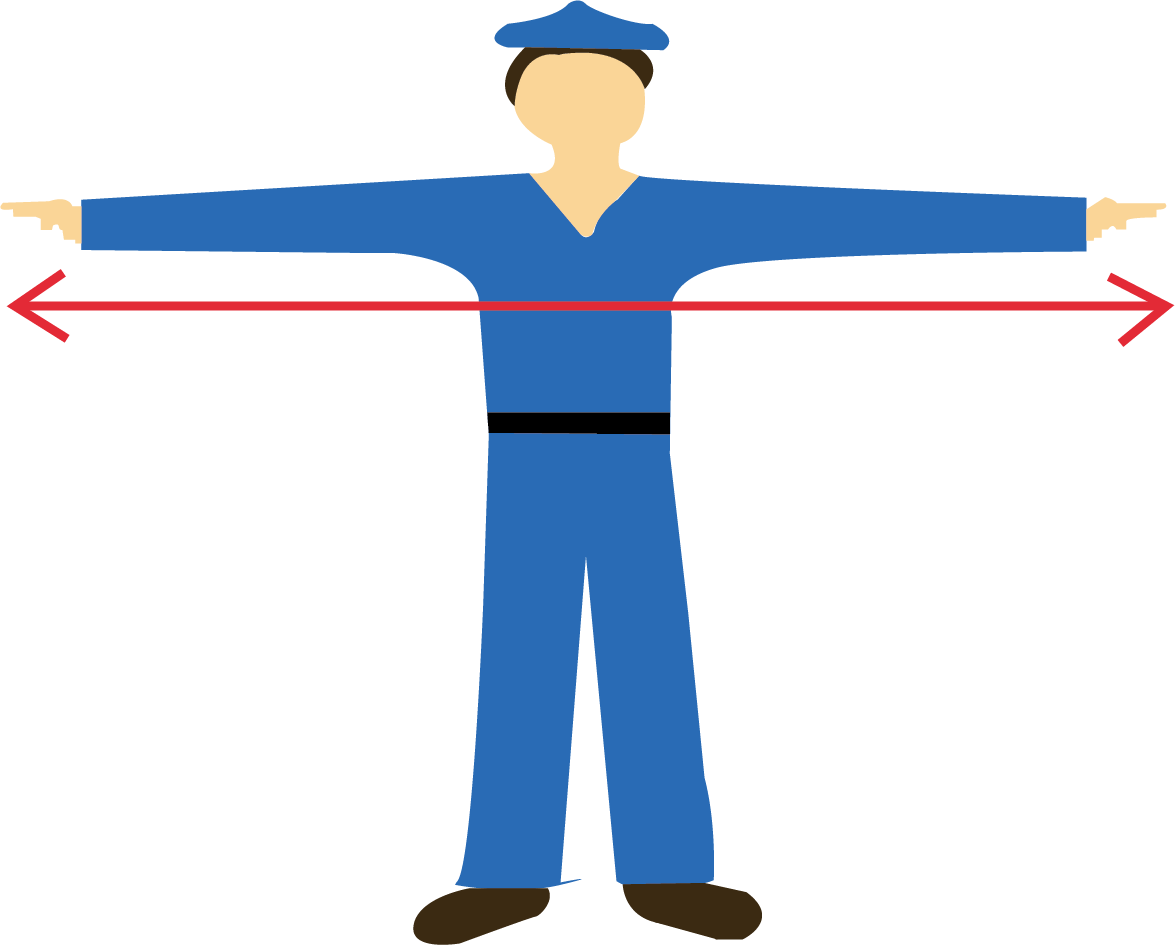Courses
Courses for Kids
Free study material
Free LIVE classes
More

# Long and Short Class 4 Notes CBSE Maths Chapter 2 (Free PDF Download)LIVE
Join Vedantu’s FREE Mastercalss

## NCERT Maths Class 4 Chapter 2 Long and Short Revision Notes

Your exam preparation will become a lot easier with the Long and Short revision notes formulated by the top subject experts at Vedantu. It is time to add these notes to your study material to get a concise understanding of the basic concepts of lines and lengths.

The revision notes for CBSE Class 4 Maths Chapter 2 Long and Short will teach you how to measure lines using a centimeter scale. It will also teach how to interconvert the length units and compare them. Download these revision notes in PDF format for free and refer to them at your convenience.

Get the free PDF version of Class 4 Maths Chapter 2 Long and Short worksheets and revision notes to complete your study. Become more efficient in answering exercise questions. Learn how the experts have explained everything mentioned in this chapter and find 5 exercise about Long and Short that you can write easily.

Last updated date: 21st Sep 2023
Total views: 144k
Views today: 4.44k

## Long and Short :

• The long and short are the adjectives or parameters to measure something.

• The two most important terms used while calculating or measuring something are “long and short”.

• These are the scales of measurement to measure distance , time , height  etc.

• Long is the word used when something measures very big. Long refers to a large value whenever something is measured.

• Short can be said as opposite to long. It refers to something that measures less or not so big.Length of Pencils Comparison

The above figure shows the length of two pencils.

• Yellow pencil is 11 units long.

• Green pencil is 9 units long.

As we know that 9 is smaller than 11. If we compare the lengths of these two pencils, then we can say that, yellow pencil is long and green pencil is short.

## Length and Distance:

• Distance is nothing but measurement of a length of some object, say, how long the road is, gives its distance. Distance is how far apart objects are, such as two cities or two points.

Units of Length and Distance : There are several units of distance and most commonly used ones are Millimetres , centimetres, metres ,Kilometres etc.

• Millimetres: Millimetres is a unit of length in the metric system. A millimetre is one thousandth of a metre. It is represented by ‘mm’.

 1000 mm = 1 mRepresentation of cm and mm on a Scale

• Centimetres: Centimetres a unit of length in the metric system, equal to one hundredth of a metre. It is denoted by ‘cm’

 100 cm = 1 mRepresentation of cm on a Scale

• Metres: Metres basic or fundamental unit of length in the metric system. It is denoted by ‘m’.

• Kilometres: Kilometres is a unit of length in the metric system, it is equal to one thousand metres. It is denoted by Km.

 1 km = 1000 m

## Solved Example 1: Look for things in your surroundings that are

(ii) Between 10 and 20 centimetres long

(iii) Less than 1 cm long.

Ans:

i. The things that are about 10 cm long are:

Tea Mug , Cell phones , Hair comb etc

ii. The things that are about 10 cm and 20 cm  long are:

Soft Drinks bottles , Dinner forks , Carrots etc.

iii. The things that are less than 1 cm long are:

Watermelon seed , a single piece of rice , a piece of daal etc.

## Solved Example 2: Convert the following into Metres

i. 700 cm

ii. 3000 mm

iii. 2 km

Ans: To convert the following into metres we need to scale between the following and metres.

i. 700 cm

We know that  100 cm = 1 m , therefore  700 cm can be obtained by multiplying 7 to both sides. i.e

$Rightarrow 7 (100 cm) = 7 (1 m)$

$Rightarrow 700 cm = 7 m.$

ii. 3000 mm

We know that 1000 mm = 1 m , therefore 3000 mm can be obtained by multiplying 3 to both sides. i.e

$Rightarrow 3 (1000 mm) = 3 (1 m)$

$Rightarrow 3000 mm = 3 m.$

iii. 2 km

We know that 1000 m = 1 km , therefore 2000 m can be obtained by multiplying 2 to both sides. i.e

$Rightarrow 2 (1000 m) = 2 (1 km)$

$Rightarrow 2 km = 2000 m.$

## How Many ?( The Art of Finding Total)

• How many simply means “what number of”.

• It is usually used to find a multiple of one thing with respect to another.

• For example, how many times Chennai Super Kings have won the IPL? Here winning IPL is the event and we are supposed to find the multiple of it.

• If we say Chennai have won the IPL 4 times , the event winning IPL is multiplied by 4.

Solved Examples 1: What is the length of rope , if the rope is 6 arms of Roopa and Roopa’s arm is  25 cm long?

Ans: Given , Length of Roopa’s arm = 25 cm.

The rope is 6 arms , which means 6 times the length of the arm.

Therefore , the length of rope = $6\times 25 = 150 cm$.

Length of the rope is 150 cm.

## Long and Short used for Comparison:

• In order to compare the length of two quantities , we need to find the length of each of them ( that is how long or short they are).

• For example, to say which is longer, thumb or middle finger? First we need to measure them and we can conclude which one is longer.

• So this goes without saying that the length of the middle finger is longer than the thumb.

• Interesting fact: For most people, their arm span is about equal to their height. Mathematicians say the arm span to height ratio is one to one: your arm span goes once into your height.Arm Span of a Human Being

Solved Examples 1: Who has a longer hand , a new born baby or an adult ?

Ans: It is quite evident and clear that an adult definitely has longer hands compared to a new born baby.

## Practice Questions:

1. The distance between two cities is measured in ?

2. Name any four objects that are 1 m long ?

1. Kilometres

2. Guitars, yard sticks , Baseball bats , Doorknob Height etc.,

## Conclusion

Vedantu's Long and Short Class 4 Notes for CBSE Maths Chapter 2 offer a valuable resource for young learners. Providing a comprehensive and concise overview of the subject, these notes cater to the specific needs of Class 4 students. The availability of a free PDF download makes it accessible to all, promoting inclusivity in education. Vedantu's commitment to delivering high-quality educational materials is evident through these notes, aiding students in grasping mathematical concepts effectively. By offering both long and short versions, students can choose the format that suits their learning style best. Overall, Vedantu's Class 4 Maths Chapter 2 notes serve as an excellent aid for young learners on their academic journey.

## FAQs on Long and Short Class 4 Notes CBSE Maths Chapter 2 (Free PDF Download)

1. Will NCERT Maths Class 4 Chapter 2 focus on different units of length?

Chapter 2 of Class 4 CBSE Maths focuses on teaching students the differences in lengths, breadths, heights, and distance using arithmetic sums. There are certain activities covered in the chapter that help students understand the basic concepts.

2.  What is the benefit of studying NCERT Class 4 Maths Chapter 2 revision notes?

The revision notes for NCERT Class 4 Maths Chapter 2 will help you to understand the concepts of length and lines easily. You will also be able to correlate the fundamentals and develop problem-solving skills to solve the sums from this chapter effectively with the help of these revision notes.

3. Are CBSE Class 4 Chapter 2 Maths revision notes available for free?

The NCERT Maths Class 4 Chapter 2 revision notes PDF is available for free download on Vedantu mobile app and official website. So you can download and refer to the revision notes PDF online and offline according to your convenience.

4. Why is learning about "Long and Short" important?

Learning about "Long and Short" is essential because measurement is a fundamental mathematical concept used in various aspects of everyday life. Understanding lengths and heights helps students compare objects, estimate sizes, and solve practical problems.

5. What are non-standard units of measurement?

Non-standard units of measurement are informal or everyday references used to measure objects. For example, using handspan, footstep, or cubit to measure lengths are considered non-standard units.